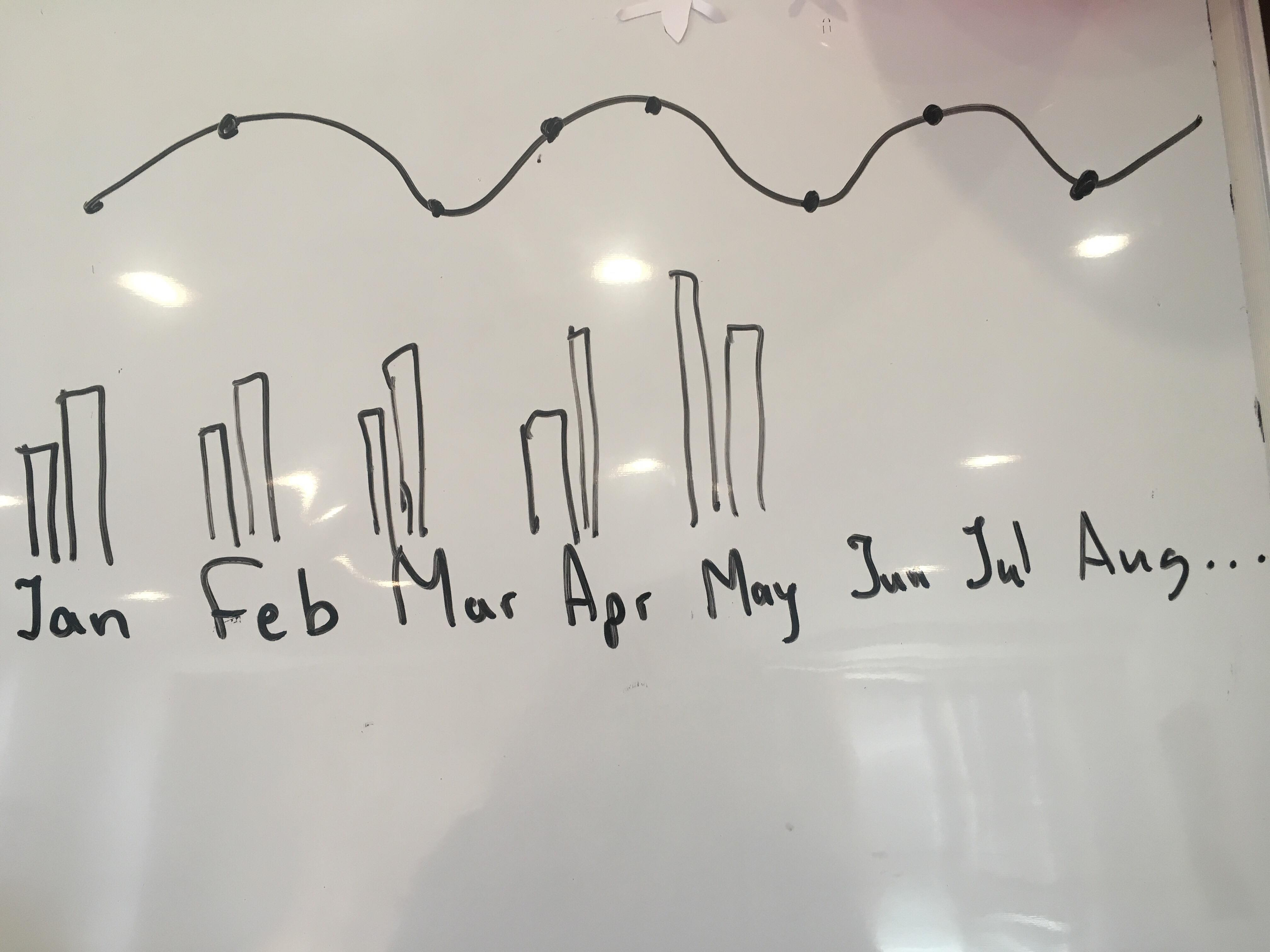# New to Qlik Sense

Discussion board where members can get started with Qlik Sense.

Highlighted
New Contributor III

## Re: Year comparison in bar chart

I cantMVP

## Re: Year comparison in bar chart

Mock up data my friend...

New Contributor III

## Re: Year comparison in bar chart

It's okay.

Can I use this formula in bar chart like this?In January second chart should be current year and  first chart should be the previous and it will show the sum for the same companies. But in this case I have 3years and I want to show all of them in all months'. I also want to have drill down  on them. When I select January , all dates  of the january should be shown and every date should have the comparison chart to the previous date( as it was in months)MVP

## Re: Year comparison in bar chart

I believe it should work, but I am new to Qlik Sense too... may be omarbensalem‌ can offer his expertise here

New Contributor III

## Re: Year comparison in bar chart

Sorry for disturbing you again, but can you tell me where should I add {1-\$} in the formulas that you told me to ignore all current selections?

And thanks a lot !!! I really appreciate your help!!MVP

## Re: Year comparison in bar chart

In this one?

=Sum({\$<Date = {">=\$(=YearStart(Today()))<=\$(=MonthEnd(Today()))"}>} FactSoldAmount)

=Sum({\$<Date = {">=\$(=YearStart(AddYears(Today(), -1)))<=\$(=MonthEnd(AddYears(Today(), -1)))"}, Company = p({<Date = {">=\$(=YearStart(Today()))<=\$(=MonthEnd(Today()))"}>})>}FactSoldAmount)

=Sum({\$<Date = {">=\$(=YearStart(AddYears(Today(), -2)))<=\$(=MonthEnd(AddYears(Today(), -2)))"}, Company = p({<Date = {">=\$(=YearStart(Today()))<=\$(=MonthEnd(Today()))"}>})>}FactSoldAmount)

May be here (I believe that just {1} should be enough not sure why you need {1-\$}...

=Sum({1<Date = {">=\$(=YearStart(Today()))<=\$(=MonthEnd(Today()))"}>} FactSoldAmount)

=Sum({1<Date = {">=\$(=YearStart(AddYears(Today(), -1)))<=\$(=MonthEnd(AddYears(Today(), -1)))"}, Company = p({1<Date = {">=\$(=YearStart(Today()))<=\$(=MonthEnd(Today()))"}>})>}FactSoldAmount)

=Sum({1<Date = {">=\$(=YearStart(AddYears(Today(), -2)))<=\$(=MonthEnd(AddYears(Today(), -2)))"}, Company = p({1<Date = {">=\$(=YearStart(Today()))<=\$(=MonthEnd(Today()))"}>})>}FactSoldAmount)

New Contributor III

## Re: Year comparison in bar chart

Yes , it works .And if I want to ignore only the year and month?  I tried to write {1} only before the first date  but it seems I'm incorrectMVP

## Re: Year comparison in bar chart

Replace 1 with <Year, Month>

New Contributor III

## Re: Year comparison in bar chart

HelloI have one question regarding this issue. I want to have climber selection bar where I can see the Companies in the years that I select.  I use the formulas that you told me in the bar chart and it works well.

=Sum({\$<Year,Date = {">=\$(=YearStart(Today()))<=\$(=MonthEnd(Today()))"}>} FactSoldAmount)

=Sum({\$<Year, Date = {">=\$(=YearStart(AddYears(Today(), -1)))<=\$(=MonthEnd(AddYears(Today(), -1)))"}, BranchID = p({<Year = {"\$(=Max(Year))"}>})>} FactSoldAmount)

=Sum({\$<Year, Date = {">=\$(=YearStart(AddYears(Today(), -2)))<=\$(=MonthEnd(AddYears(Today(), -2)))"}, BranchID = p({<Year = {"\$(=Max(Year))"}>})>}FactSoldAmount)

In the climber bar I have company in dimension and it shows me all companies. But I want to show only the companies in these years.  And when I click years it works well. Can you help me ?MVP

## Re: Year comparison in bar chart

Climber bar? I am not sure I know what that is